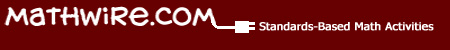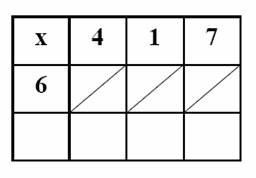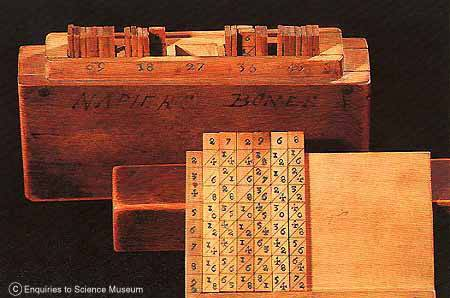Alternate Multiplication Algorithms

Students today develop proficiency with many different algorithms for multiplication. This approach insures that each student will find a method that works effectively for him/her. Teachers model the different algorithms and encourage students to use and practice each method before selecting a favorite.## Lattice Multiplication

This algorithm works well for students who are developing multiplication fact fluency. Students may begin using a template to solve multiplication problems, but they quickly learn to draw their own lattice matrix to solve problems. Students love the method and it is very successful with both whole numbers and decimals. Teach students this multiplication algorithm as one of many different algorithms they may elect to use.Procedure:

• Student writes the problem in the grid (e.g. 6 x 417).
• Student then writes the answer to each single digit multiplication in the appropriate square.
• The tens digit is placed above the diagonal; the ones digit below the diagonal.
• When all squares have been completed, the student sums the numbers between each set of diagonals, and writes the sum at the bottom of the grid. NOTE: If the sum is greater than 10, regroup the ten to the next diagonal to the left.
• The student can now simply read the answer from left to right and insert commas, as appropriate.

Practice:

Templates:   Three different templates are included for Lattice Multiplications.   Templates are designed to be used in sheet protectors.   This strategy allows students to use dry-erase markers and re-use the same paper for many multiplication problems.

• Download theLattice grids for a single digit multiplier.
• Download theLattice grid for a 2-digit multiplier.
• Download theLattice grid for 2-3 digit multipliers.## Napier's Bones

Once students are comfortable with lattice multiplication, Napier's Bones is a great enrichment activity. Students order the bones as they would write the problem in the lattice. They are then able to read the answer without any writing. It's magic!

• DownloadNapier's Bones template that students may cut apart to create the bones. The file contains three different versions including blank bones that students fill in to create the bones, developing a richer understanding of the magic of the bones. Copy the handout on card stock and laminate before cutting to create more durable classroom sets.•Cool Math for Kids Lattice Multiplication is a colorful and simple presentation of Lattice Multiplication that would be a great introduction for younger students and an effective resource for parents as well.
•Lattice Multiplication Slide Show that explains the process step-by-step.
•Lattice Multiplication history and step-by-step illustrated directions.
•Smart Board Templates for Lattice Multiplication and many other math applications.
• See Wolfram MathWorld'sNapier's Bones for an explanation of the process.
•Bones Multiplication Technique is a flash presentation that includes a demo and the ability to input a problem to multiply. This resource is designed for older students and adults.
• SeePicture of Napier's Bones.